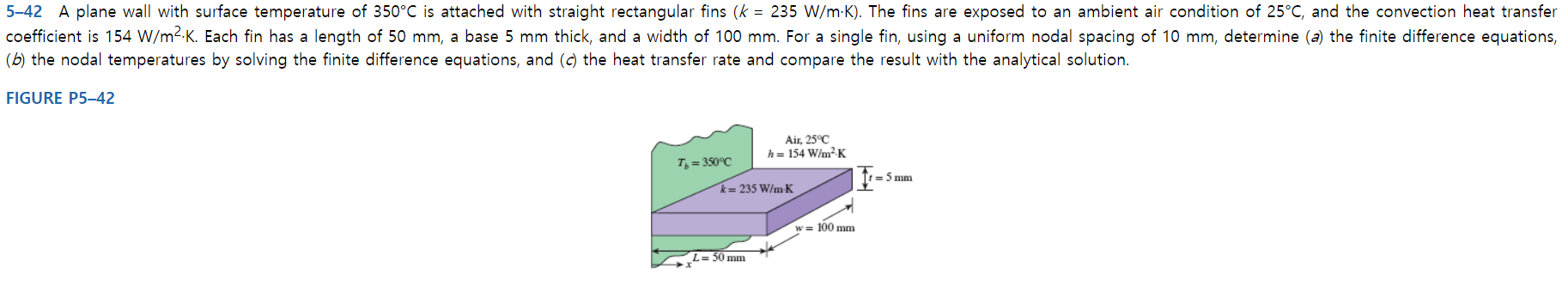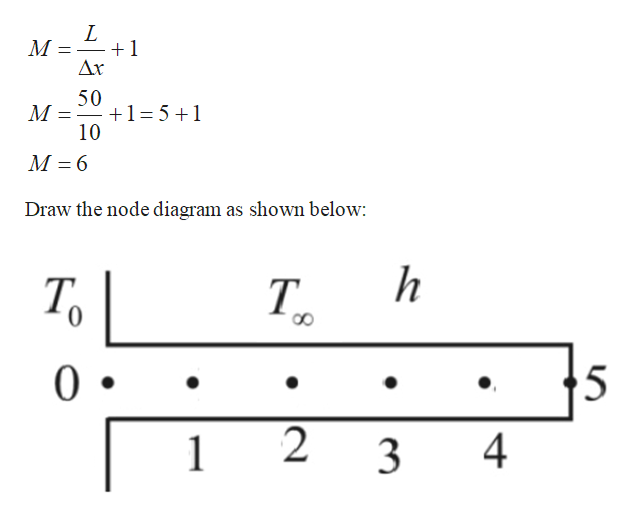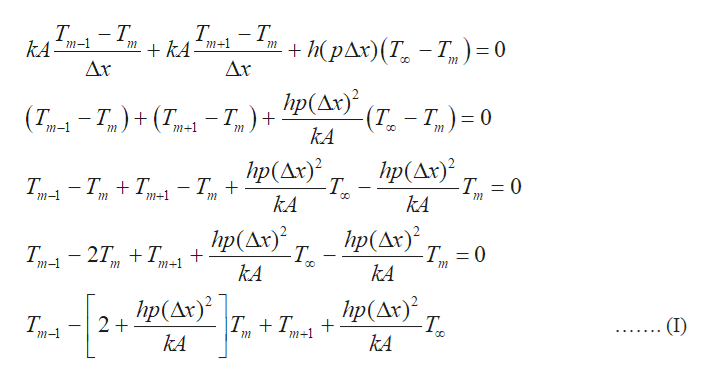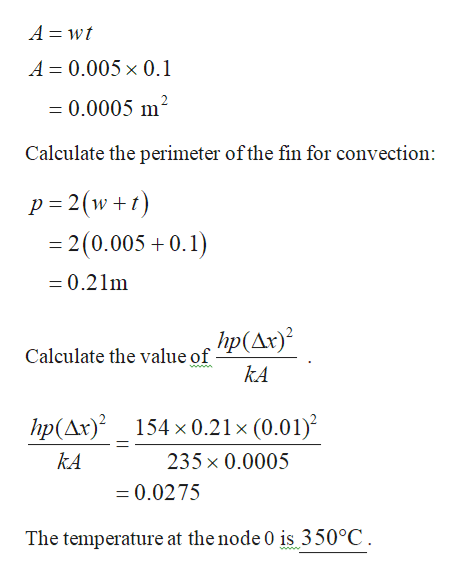# 5-42 A plane wall with surface temperature of 350°C is attached with straight rectangular fins (k 235 W/m K). The fins are exposed to an ambient air condition of 25°C, and the convection heat transfercoefficient is 154 W/m2-K. Each fin has a length of 50 mm, a base 5 mm thick, and a width of 100 mm. For a single fin, using a uniform nodal spacing of 10 mm, determine (a) the finite difference equations,(b) the nodal temperatures by solving the finite difference equations, and (c) the heat transfer rate and compare the result with the analytical solution.FIGURE P5-42Air, 25°Ch= 154 W/m2KT,-350°C=5 mmk= 235 W/m Kw= 100 mmL=50 mm

Question
9 viewshelp_outlineImage Transcriptionclose5-42 A plane wall with surface temperature of 350°C is attached with straight rectangular fins (k 235 W/m K). The fins are exposed to an ambient air condition of 25°C, and the convection heat transfer coefficient is 154 W/m2-K. Each fin has a length of 50 mm, a base 5 mm thick, and a width of 100 mm. For a single fin, using a uniform nodal spacing of 10 mm, determine (a) the finite difference equations, (b) the nodal temperatures by solving the finite difference equations, and (c) the heat transfer rate and compare the result with the analytical solution. FIGURE P5-42 Air, 25°C h= 154 W/m2K T,-350°C =5 mm k= 235 W/m K w= 100 mm L=50 mm fullscreen
check_circle

Step 1

Calculate the number of nodes:help_outlineImage TranscriptioncloseМ 1 Ax 50 1 51 10 М -6 Draw the node diagram as shown below: Т. 5 0 2 4 1 3 fullscreen
Step 2

Write the general finite difference relation for each node:help_outlineImage TranscriptioncloseT Т. kA Т. m+1 kA T + КрАr)(T, — Т, Ar m-1 = (0 CO Дr Пр(Дк)? Т,) %3D0 (Т, - Т,) + (Т. —т,) + — Т.) + (T. m+1 (Т. kA т-1 Пp(Дr)? -Т, Пp(Дr)? Т, -T 0 — Т, + Т. m-1 m+1 kA kA hp(Ar) -То Ip(Aк)? -T 0 Т, — 27, + Т, m-1 m+1 kKA kA hp(Ax) hp(Ar) 2+ |T+T (I) m-1 m+1 kA kA fullscreen
Step 3

Calculate the area of fi...help_outlineImage TranscriptioncloseA wt A 0.005 x 0.1 =0.0005 m2 Calculate the perimeter ofthe fin for convection: p 2(w = 2 (0.005+0.1) +t =0.21m hp(Ar)? Calculate the value of ww. kA hp(Ar)2 154 x0.21 x (0.01) kA 235x 0.0005 =0.0275 The temperature at the node 0 is 350°C fullscreen

### Want to see the full answer?

See Solution

#### Want to see this answer and more?

Solutions are written by subject experts who are available 24/7. Questions are typically answered within 1 hour.*

See Solution
*Response times may vary by subject and question.
Tagged in

### Mechanical Engineering